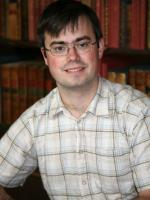# Faculty of MathematicsProfessor (Grade 11) in Number Theory

Research Interests: My research interests are in arithmetical algebraic geometry and computational number theory. In particular I work on elliptic curve descent calculations, and the construction of explicit elements in the Tate-Shafarevich group.

## Publications

Higher descents on an elliptic curve with a rational 2-torsion point
T Fisher
– Mathematics of Computation
(2017)
86,
2493
On genus one curves of degree 5 with square-free discriminant
– JOURNAL OF THE RAMANUJAN MATHEMATICAL SOCIETY
(2016)
31,
359
Visualizing elements of order $7$ in the Tate–Shafarevich group of an elliptic curve
T Fisher
– LMS Journal of Computation and Mathematics
(2016)
19,
100
The proportion of plane cubic curves over Q that everywhere locally have a point
M Bhargava, J Cremona, T Fisher
– International Journal of Number Theory
(2016)
12,
1077
What is the Probability that a Random Integral Quadratic Form in n Variables has an Integral Zero?
M Bhargava, JE Cremona, T Fisher, NG Jones, JP Keating
– International Mathematics Research Notices
(2015)
2016,
3828
Ranks of quadratic twists of elliptic curves
M Watkins, S Donnelly, ND Elkies, T Fisher, A Granville, NF Rogers
– Publications Mathématiques de Besançon
(2015)
63
(doi: 10.5802/pmb.9)
On families of 9-congruent elliptic curves
T Fisher
– Acta Arithmetica
(2015)
171,
371
Computing the Cassels-Tate pairing on the 3-Selmer group of an elliptic curve
T Fisher, R Newton
– International Journal of Number Theory
(2014)
10,
1881
On families of 7- and 11-congruent elliptic curves
T Fisher
– LMS Journal of Computation and Mathematics
(2014)
17,
536
Minimal models for $\def \xmlpi #1{}\def \mathsfbi #1{\boldsymbol {\mathsf {#1}}}\let \le =\leqslant \let \leq =\leqslant \let \ge =\geqslant \let \geq =\geqslant \def \Pr {\mathit {Pr}}\def \Fr {\mathit {Fr}}\def \Rey {\mathit {Re}}6$-coverings of elliptic curves
T Fisher
– LMS Journal of Computation and Mathematics
(2014)
17,
112
• <
• 2 of 5
• >

## Research Group

Number Theory & Algebra

E1.09

01223 764275